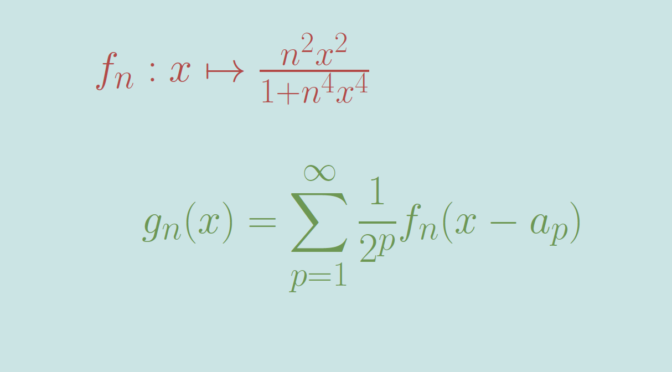# Pointwise convergence not uniform on any interval

We provide in this article an example of a pointwise convergent sequence of real functions that doesn’t converge uniformly on any interval.

Let’s consider a sequence $$(a_p)_{p \in \mathbb N}$$ enumerating the set $$\mathbb Q$$ of rational numbers. Such a sequence exists as $$\mathbb Q$$ is countable.

Now let $$(g_n)_{n \in \mathbb N}$$ be the sequence of real functions defined on $$\mathbb R$$ by $g_n(x) = \sum_{p=1}^{\infty} \frac{1}{2^p} f_n(x-a_p)$ where $$f_n : x \mapsto \frac{n^2 x^2}{1+n^4 x^4}$$ for $$n \in \mathbb N$$.

### $$f_n$$ main properties

$$f_n$$ is a rational function whose denominator doesn’t vanish. Hence $$f_n$$ is indefinitely differentiable. As $$f_n$$ is an even function, we can study it only on $$[0,\infty)$$.

We have $f_n^\prime(x)= 2n^2x \frac{1-n^4x^4}{(1+n^4 x^4)^2}.$ $$f_n^\prime$$ vanishes at zero (like $$f_n$$) is positive on $$(0,\frac{1}{n})$$, vanishes at $$\frac{1}{n}$$ and is negative on $$(\frac{1}{n},\infty)$$. Hence $$f_n$$ has a maximum at $$\frac{1}{n}$$ with $$f_n(\frac{1}{n}) = \frac{1}{2}$$ and $$0 \le f_n(x) \le \frac{1}{2}$$ for all $$x \in \mathbb R$$.

Also for $$x \neq 0$$ $0 \le f_n(x) =\frac{n^2 x^2}{1+n^4 x^4} \le \frac{n^2 x^2}{n^4 x^4} = \frac{1}{n^2 x^2}$ consequently $0 \le f_n(x) \le \frac{1}{n} \text{ for } x \ge \frac{1}{\sqrt{n}}.$

### $$(g_n)$$ converges pointwise to zero

First, one can notice that $$g_n$$ is well defined. For $$x \in \mathbb R$$ and $$p \in \mathbb N$$ we have $$0 \le \frac{1}{2^p} f_n(x-a_p) \le \frac{1}{2^p} \cdot\ \frac{1}{2}=\frac{1}{2^{p+1}}$$ according to previous paragraph. Therefore the series of functions $$\sum \frac{1}{2^p} f_n(x-a_p)$$ is normally convergent. $$g_n$$ is also continuous as for all $$p \in \mathbb N$$ $$x \mapsto \frac{1}{2^p} f_n(x-a_p)$$ is continuous.

Now fix $$x \in \mathbb R$$. For $$0< \epsilon < \frac{1}{2}$$ we denote $$p_\epsilon = \sup\{p \in \mathbb N \ ; \ \frac{1}{2^p} > \epsilon\}$$ and $$A_\epsilon= \{a_p \ ; \ p \le p_\epsilon\}$$. $$A_\epsilon$$ is a finite subset of reals, hence the distance $$\delta = d(x,A_\epsilon \setminus \{x\})$$ is strictly positive. Denoting $$N=\lceil \sup(\frac{1}{\epsilon},\frac{1}{\delta^2}) \rceil$$, we have for $$n \ge N$$$\vert x – a_p \vert \ge \delta \ge \frac{1}{\sqrt{n}} \text{ for } a_p \in A_\epsilon \setminus \{x\}$ and therefore $$0 \le f_n(x-a_p) \le \frac{1}{n} \le \epsilon$$ for $$a_p \in A_\epsilon$$. Finally for $$n \ge N$$\begin{aligned} 0 &\le g_n(x) = \sum_{p=1}^{\infty} \frac{1}{2^p} f_n(x-a_p)\\ &=\sum_{p=1}^{p_\epsilon} \frac{1}{2^p} f_n(x-a_p) + \sum_{p=p_\epsilon+1}^\infty \frac{1}{2^p} f_n(x-a_p)\\ &\le \sum_{p=1}^{p_\epsilon} \frac{1}{2^p} \epsilon + \sum_{p=p_\epsilon+1}^\infty \frac{1}{2^p} \frac{1}{2}\\ &\le \epsilon\sum_{p=1}^\infty \frac{1}{2^p} + \frac{1}{2}\sum_{p=p_\epsilon+1}^\infty \frac{1}{2^p}\\ &\le \epsilon\sum_{p=1}^\infty \frac{1}{2^p} + \frac{1}{2}\sum_{p=p_\epsilon+1}^\infty \frac{1}{2^p}\\ &= \epsilon + \frac{1}{2^{p_\epsilon+1}} \le 2 \epsilon.\\ \end{aligned} This concludes the proof that $$(g_n)$$ converges pointwise to zero. An alternate proof can be based on Lebesgue’s dominated convergence theorem using the discrete measure $$\displaystyle \sum_{p \in \mathbb N} \frac{1}{2^p} \delta_{a_p}$$ where $$\delta_x$$ denotes the Dirac measure centered on some fixed point $$x$$.

### $$(g_n)$$ doesn’t converge uniformly on any open interval

Consider an open interval $$(u,v)$$ with $$u < v$$. As $$\mathbb Q$$ is dense in $$\mathbb R$$, it exists $$q \in \mathbb N$$ such that $$a_q \in (u,v)$$. As $$(u,v)$$ is open, we also have $$a_q + 1/n \in (u,v)$$ for $$n$$ large enough. Then $g_n(a_q+1/n) \ge \frac{1}{2^q} f_n(a_q+1/n-a_q)=\frac{1}{2^q}f_n(1/n)=\frac{1}{2^{q+1}}$ proving that $$(g_n)$$ doesn't converge uniformly on $$(u,v)$$.